# All commands, alphabetic order; letter V

## \v

The \v command puts a hacheck accent on some letters. These are the possibilities:


\v A \v a \v C \v c \v D \v d \v E \v e \v G \v g
\v H \v h \v K \v k \v I \v i \v j \v L \v l \v N
\v n \v O \v o \v R \v r \v S \v s \v T \v t \v U
\v u  \v{\"U} \v{\"u} \v{\.S} \v{\.s} \v Z \v z


Translation is the following sequence of characters Ǎ ǎ Č č Ď ď Ě ě Ǧ ǧ Ȟ ȟ Ǩ ǩ Ǐ ǐ ǰ Ľ ľ Ň ň Ǒ ǒ Ř ř Š š Ť ť Ǔ ǔ Ǚ ǚ Ṧ ṧ Ž ž

&#461; &#462; &#268; &#269; &#270; &#271; &#282; &#283; &#486; &#487;
&#542; &#543; &#488; &#489; &#463; &#464; &#496; &#317; &#318; &#327;
&#328; &#465; &#466; &#344; &#345; &#352; &#353; &#356; &#357; &#467;
&#468; &#473; &#474; &#7782; &#7783; &#381; &#382;


## \V (Tralics command)

The command \V produces a check under accent. Example.

\V D \V d \V E \V e \V L \V l \V N \V n \V T \V t \V U \V u


Translation is the following sequence of characters Ḓ ḓ Ḙ ḙ Ḽ ḽ Ṋ ṋ Ṱ ṱ Ṷ ṷ

&#7698; &#7699; &#7704; &#7705; &#7740; &#7741; &#7754;
&#7755; &#7792; &#7793; &#7798; &#7799;


Unimplemented; see here for details.

## \valign

This is like \halign, not implemented.

## \value

You say \value{foo} when you want the value of the counter named foo. In fact, \value{foo} expands to \c@foo, with a check that the counter exists.

## \varbigcirc (math symbol)

The \varbigcirc command is valid only in math mode. It is an alias for \bigcirc.

## \varbigtriangledown (math symbol)

The \varbigtriangledown command is valid only in math mode. It is an alias for \bigtriangledown.

## \varbigtriangleup (math symbol)

The \varbigtriangleup command is valid only in math mode. It is an alias for \bigtriangleup.

## \varcurlyvee (math symbol)

The \varcurlyvee command is valid only in math mode. It is an alias for \curlyvee.

## \varcurlywedge (math symbol)

The \varcurlywedge command is valid only in math mode. It is an alias for \curlywedge.

## \varepsilon (math character)

The \varepsilon command is valid only in math mode. It generates a Greek letter: <mi>&varepsilon;</mi> (Unicode U+03B5, ε).

## \varinjlim (math command)

The \varinjlim command is a variant of \injlim. It behaves like \lim, where the operator is lim with a right arrow below it. For instance the translation of

$\varinjlim _x < \varprojlim_y, \injlim_X <\projlim_ Y$


will be

<formula type='display'>
<math mode='display' xmlns='http://www.w3.org/1998/Math/MathML'>
<mrow>
<munder>
<munder accentunder='true'>
<mo movablelimits='false'>lim</mo>
<mo>&rightarrow;</mo>
</munder>
<mi>x</mi>
</munder>
<mo>&lt;</mo>
<munder>
<munder accentunder='true'>
<mo movablelimits='false'>lim</mo>
<mo>&leftarrow;</mo>
</munder>
<mi>y</mi>
</munder>
<mo>,</mo>
<munder>
<mo movablelimits='true' form='prefix'>inj lim</mo>
<mi>X</mi>
</munder>
<mo>&lt;</mo>
<munder>
<mo movablelimits='true' form='prefix'>proj lim</mo>
<mi>Y</mi>
</munder>
</mrow>
[/itex]
</formula>


## \varkappa (math character)

The \varkappa command is valid only in math mode. It generates a Greek letter: <mi>&kappav;</mi> (Unicode U+03F0, ϰ).

## \varliminf (math command)

The \varliminf command is a variant of \liminf. It behaves like \lim, where the operator is an underlined lim. For instance the translation of

$\varliminf _x < \varlimsup_y, \liminf_X <\limsup_ Y$


will be

<formula type='display'>
<math mode='display' xmlns='http://www.w3.org/1998/Math/MathML'>
<mrow>
<munder>
<munder><mo movablelimits='false'>lim</mo> <mo>&UnderBar;</mo></munder>
<mi>x</mi>
</munder>
<mo>&lt;</mo>
<munder>
<mover><mo movablelimits='false'>lim</mo> <mo>&OverBar;</mo></mover>
<mi>y</mi>
</munder>
<mo>,</mo>
<munder>
<mo movablelimits='true' form='prefix'>lim inf</mo>
<mi>X</mi>
</munder>
<mo>&lt;</mo>
<munder>
<mo movablelimits='true' form='prefix'>lim sup</mo>
<mi>Y</mi>
</munder>
</mrow>
[/itex]
</formula>


## \varlimsup (math command)

The \varlimsup command is a variant of \limsup. It behaves like \lim, where the operator is an overlined lim. See example above.

## \varnothing (math symbol)

The \varnothing command is valid only in math mode. Its translation is: <mi>&#x2300;</mi>, ⌀.

## \varoast (math symbol)

The \varoast command is valid only in math mode, it is an alias to \oast.

## \varobar (math symbol)

The \varobar command is valid only in math mode, an alias to \obar.

## \varoblash (math symbol)

The \varobslash command is valid only in math mode, it is an alias to \oslash.

## \varocircle (math symbol)

The \varocircle command is valid only in math mode. Its translation is <mo>&#x29BE;</mo>, ⦾.

## \varodot (math symbol)

The \varodot command is valid only in math mode, it is an alias to \odot.

## \varogreaterthan (math symbol)

The \varogreaterthan command is valid only in math mode, it is an alias to \ogreaterthan.

## \varolessthan (math symbol)

The \varolessthan command is valid only in math mode, it is an alias to \olessthan.

## \varominus (math symbol)

The \varominus command is valid only in math mode, it is an alias to \ominus.

## \varoplus (math symbol)

The \varoplus command is valid only in math mode, it is an alias to \oplus.

## \varoslash (math symbol)

The \varoslash command is valid only in math mode, it is an alias to \oslash.

## \varotimes (math symbol)

The \varotimes command is valid only in math mode, it is an alias to \otimes.

## \varphi (math symbol)

The \varphi command is valid only in math mode. It generates a Greek letter: <mi>&phi;</mi> (Unicode character U+3C6, φ).

## \varpi (math symbol)

The \varpi command is valid only in math mode. It generates a Greek letter: <mi>&piv;</mi> (Unicode character U+3D6, ϖ).

## \varprime (math symbol)

The \varprime command is valid only in math mode. It generates <mo>&prime;</mo> (Unicode character U+2032, ′), this is a prime character (not supposed to be used as an exponent like \prime).

## \varprojlim (math command)

The \varprojlim command is a variant of \projlim. It behaves like \lim, where the operator is lim with a left arrow. For an example, see \varinjlim.

## \varrho (math symbol)

The \varrho command is valid only in math mode. It generates a Greek letter: <mi>&rhov;</mi> (Unicode character U+3F1, ϱ).

## \varsigma (math symbol)

The \varsigma command is valid only in math mode. It generates a Greek letter: <mi>&sigmav;</mi> (Unicode character U+3C2, ς).

## \vartheta (math symbol)

The \vartheta command is valid only in math mode. It generates a Greek letter: <mi>&thetav;</mi> (Unicode character U+3D1, ϑ).

## \vartimes (math symbol)

The \vartimes command is valid only in math mode, it is an alias to \times.

## \vartriangleleft (math symbol)

The \vartriangleleft command is valid only in math mode. It generates <mo>&vartriangleleft;</mo> (Unicode character U+22B2, ⊲).

## \vartriangleright (math symbol)

The \vartriangleright command is valid only in math mode. It generates <mo>&vartriangleright;</mo> (Unicode character U+22B3, ⊳).

If you say \vbadness=26, then TeX will show all vboxes with badness greater than 26. This parameter is ignored by Tralics, since no badness is computed. (See scanint for details of argument scanning).

## \vbox

You can say \vbox to 3cm{xx} or \vbox spread 3cm{xx} or simply \vbox{xx} (see scandimen for how dimensions are read). The effect is the same: it translates the xx locally in a group and puts in an anonymous box (the result is <>xx</> and this is printed as xx). The result can be used as a box for other operations.

X\setbox0=\vsplit 25 to 4cm \box0Y.
X\setbox0=\vtop to 2cm{x}\box0Y.
X\setbox0=\vbox to 2cm{x}\box0Y.
X\setbox0=\hbox to 2cm{x}\box0Y.


Translation

XY.
XxY.
XxY.
XxY.
XxY.
XxY.
XxY.


## \vcenter

The \vcenter command is valid only in math mode. A vbox is formed as if \vcenter had been \vbox. Then a new Vcent atom is appended to the current math list, and the box becomes its nucleus. (Citation from the TeXbook, Chapter 26).

In Tralics an error is signaled, because of limitations of MathML. As a consequence, the command is allowed only outside math mode, and behaves like \vbox. For instance \vcenter to 5pt {\bf toto} creates an anonymous element contains toto' in bold-face font.

## \vdash, \vDash, \Vdash, \VDash (math symbol)

The \vdash command is valid only in math mode. It generates a relation symbol: <mo>&vdash;</mo> This is the Unicode character U+22A2, ⊢; there is a variant U+22A6 ⊦ that looks the same. The command \vDash is similar, it produces character U+22A7, ⊧ it has a double horizontal bar; the command \Vdash is similar, it produces character U+22A9 ⊩, it has a double vertical bar; finally \VDash produces character U+22AB, ⊫ that has a double vertical bar, and a double horizontal bar. These commands can be negated via \nvdash, \nvDash, \nVdash, \nVDash.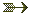See description of the \le command.

## \vdots (math symbol)

The \vdots command is valid only in math mode. It generates a miscellaneous symbol: <mo>&vellip;</mo> (Unicode U+22EE, ⋮) .See description of the \ldots command.

Note: since this is defined in LaTeX by \def\vdots{\vbox{\baselineskip4\p@ \lineskiplimit\z@ \kern6\p@\hbox{.}\hbox{.}\hbox{.}}}, we allow it outside math mode, translation is a math formula in this case.

## \vec

The \vec command puts a vector accent over a kernel. It works only in math mode.See also the \acute command.

## \vector

You say \vector(dx,dy){length} in order to put a straight line (with an arrow tip) into a picture. See description of the picture environment for the syntax of the (dx,dy) part, and some examples. In general, the \vector is the argument of a \put(x,y){} command, and the (x,y) positions indicate the starting point of the line segment; the arrow head is at the opposite end. Quantities dx and dy should be small integers (at most four in magnitude) and coprime (in fact, Tralics really does not care). If dx=0, then length is the length of the line; otherwise, length is the length of the horizontal projection of the line.

## \vee (math symbol)

The \vee command is valid only in math mode. It generates a binary operator: <mo>&vee;</mo> (Unicode character U+2228, ∨).

## \veebar (math symbol)

The \veebar command is valid only in math mode. It generates the operator: <mo>&veebar;</mo> (Unicode character U+22BB, ⊻).

## \verb

Translation of \verb+foo+ or \verb*+foo+ is the same as \verbprefix{\verbatimfont foo}. Any character can be used instead of the Plus sign as delimiter. Any character between the two delimiters (include backslash, brace, dollar signs etc) are considered as normal characters. Translation of a space is a non-break space in the non-starrred version, and a visible space character otherwise. Example

Verbatim test

\verb-\verb+ { } $$-. \verb*-\verb+ { }$$-.
\let\verbatimfont\it
\verb +x+ !


The translation is

<p>Verbatim test</p>
<p><hi rend='tt'>\verb+&#xa0;{&#xa0;}&#xa0;$$</hi>. <hi rend='tt'>\verb+&#x2423;{&#x2423;}&#x2423;$$</hi>.
<hi rend='it'>+x+</hi>!</p>


Preview: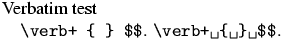Look carefully at the last example. The character that follows the \verb command is a space, and this space plays the role of the delimiter. Remember that spaces at end of line are removed, so that the exclamation point at the end of the line cannot be removed.

## verbatim (environment)

The verbatim environment lets you enter text in a mode where special characters like braces, dollars and backslashes are not interpreted. Characters like less-than, greater-than, and ampersand are escaped. Do not confuse with the rawxml environment, for which these characters are not escaped.

\begin{verbatim}
{\let\rm\bf \bf toto}
<!--this is a comment -->
&dollar; not &equals; EURO &excl;
\end{verbatim}

\begin{rawxml}
{\let\rm\bf \bf toto}
<!--this is a comment -->
&dollar; not &equals; EURO &excl;
\end{rawxml}


Here is the translation. By default, in verbatim mode, spaces are translated into character U+A0 (non-break space) that looks like a space. As you can see, Tralics inserts from time to time a <zws/> element; this is done to inhibit ligatures, for instance between two dashes. You can inhibit this behavior by calling Tralics with the option -nozerowidthspace. You can use the option -nozerowidthelt, in this case the element is replaced by the zero-width space character U+200B.

<p noindent='true'><hi rend='tt'>{\let\rm\bf&#xa0;\bf&#xa0;toto}</hi></p>
<p noindent='true'><hi rend='tt'>&lt;<zws/>!-<zws/>-<zws/>this&#xa0;is&#xa0;a
&#xa0;comment&#xa0;-<zws/>-<zws/>&gt;<zws/></hi></p>
<p noindent='true'><hi rend='tt'>&amp;dollar;&#xa0;not&#xa0;&amp;equals;&#xa0;EURO
&#xa0;&amp;excl;</hi></p>
{\let\rm\bf \bf toto}
<!--this is a comment -->
&dollar; not &equals; EURO &excl;


Preview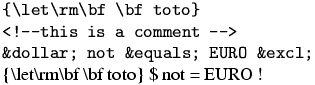See also: rawxml environment and \fvset command (this explains how spaces are translated in verbatim mode). See also Verbatim below for how fonts are chosen.

Note: The end of a verbatim-like environment is defined by a line that contains: optional spaces, the token \end, optional spaces, the name of the environment in braces (for instance the tokens {verbatim}), optional spaces, and nothing else. A line that does not match this pattern is part of the verbatim-like environment. The syntax changed in version 2.10.7: the nothing else has been removed. If anything remains on the line, it is assumed to be on a line that follows the environment. Example.

  \begin {verbatim}
This is a verbatim_line %
\end {verbatim}OK?


Translation, with no-break space character shown as entities

<p noindent='true'><hi rend='tt'>This&#xa0;is&#xa0;a&#xa0;verbatim_line&#xa0;%</hi></p>
<p noindent='true'>OK?</p>


## Verbatim (environment)

The Verbatim environment is an extension of the verbatim environment. There is an optional argument, an association list. If you say numbers=true, then lines will be numbered, starting from 1. If you say numbers=N, where N is a number, then the counter number N will be used; instead of a number, you can also give a counter name.

If a line number M is given, the following piece of code is inserted before the verbatim line: {\verbatimnumberfont{M}}\space. The funny command is \let equal to \small at the start of the run.

The line itself is evaluated via \verbatimprefix{\verbatimfont theline}, where the second funny command is \let equal to \tt at the start of the run. In the example that follows, the redefinition is not good.

Some features defined by the fancyvrb package has not yet been implemented.

Characters after \begin{Verbatim}, but on the same line, are ignored. The same is true if an optional argument is given: all characters that follow the closing bracket of the optional argument are ignored. The opening bracket is only looked for on the current line (unless the end of line character is commented out).

Example

Verbatim env with line numbers
\begin{Verbatim}                   [numbers=true]
TEST
\end{Verbatim}
and without
\begin{Verbatim}
[ok]TEST
\end{Verbatim}
\begin{Verbatim} %
[ok] this is handled as comment
TEST
\end{Verbatim}

\def\verbatimfont#1{{\it #1}}
\def\verbatimnumberfont{\large}
\tracingall
\count3=4
\begin{Verbatim}[counter=3]
5,one line
\end{Verbatim}
\begin{Verbatim}[counter=03]
6,one line
\end{Verbatim}
\newcounter{vbcounter}
\setcounter{vbcounter}8
\begin{Verbatim}[counter=vbcounter]
9,one line
\end{Verbatim}
\begin{Verbatim}[counter=vbcounter]
10,one line
\end{Verbatim}

<p>Verbatim env with line numbers</p>
<p noindent='true'><hi rend='small1'>1</hi> <hi rend='tt'>TEST</hi></p>
<p noindent='true'>and without</p>
<p noindent='true'><hi rend='tt'>[ok]TEST</hi></p>
<p noindent='true'><hi rend='tt'>TEST</hi></p>
<p noindent='true'><hi rend='large1'>5</hi> <hi rend='it'>5</hi>,one line</p>
<p noindent='true'><hi rend='large1'>6</hi> <hi rend='it'>6</hi>,one line</p>
<p noindent='true'><hi rend='large1'>9</hi> <hi rend='it'>9</hi>,one line</p>
<p noindent='true'><hi rend='large1'>10</hi> <hi rend='it'>1</hi>0,one line</p>


Preview: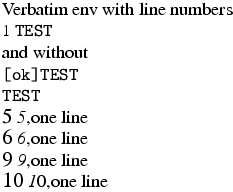Two additional keywords style and pre have been added. If you say style=foo, then the token \FV@style@foo is added in front of the token list generated by the verbatim environment. If you say pre=bar, then \FV@pre@bar is added before the token list (and before the style token mentioned above), and \FV@post@bar is inserted near the end (to be precise: before the last \par or \par\noindent. The fancyvrb package defines the value associated to style=latex and pre=bar as follows

\def\FV@pre@pre{\begin{xmlelement*}{pre}}
\def\FV@post@pre{\end{xmlelement*}}


Then the translation of

\begin{Verbatim}[pre=pre,style=latex,numbers=true]
first line
second line
\end{Verbatim}
third line


will be

<pre class='latex-code'>
<p noindent='true'> <hi rend='small'>1</hi> <hi rend='tt'>first line</hi></p>
<p noindent='true'> <hi rend='small'>2</hi> <hi rend='tt'>second line</hi></p>
</pre><p noindent='true'>third line</p>


## \verbatimfont

This is a command, defined by Tralics, that holds the command used to typeset verbatim material (either verbatim environment, or \verb command). An example of use is shown above. The initial value of the command is \tt.

## \verbatimnumberfont

This is a command, defined by Tralics, that holds the command used to typeset line numbers for verbatim material (Verbatim environment). An example of use is shown above. The initial value of the command is \small.

## \verbatimprefix

This is a command, defined by Tralics, that holds the command used to typeset verbatim environment; see below. The initial value of the command is \empty.

## \verbprefix

This is a command, defined by Tralics, that holds the command used to typeset verbatim commands. The initial value of the command is \empty.

Each line of a verbatim environment, or each verbatim word, is typeset using \verbatimfont; this command takes no argument. You can use \verbatimprefix and \verbprefix, a command that takes as argument the augmented line. There are two different commands, so that you can, for instance, typeset environments in a smaller font.


\DefineShortVerb{\|}
\def\verbatimfont#1{{#1}}
\def\verbprefix#1{A#1A}
\def\verbatimprefix#1{B#1B}
Test: \verb+foo+ and |bar|
\UndefineShortVerb{\|}
\begin{verbatim}
line1
line2
\end{verbatim}


The translation is:

<p>Test: AfooA and  AbarA</p>
<p noindent='true'>Bline1B</p>
<p noindent='true'>Bline2B</p>


## \vert (math symbol)

The \vert command is valid only in math mode. It generates a delimiter (vertical bar), <mo>&vert;</mo> (Unicode U+7C, |)

The delimiters recognized are


$\def\kernel{\frac{x^1_2}{y^3_4}} \left\Vert\left|\left[\left(\left\{ \kernel \right\}\right)\right]\right|\right\Vert \left\vert\left|\left<\left\langle \kernel \right\rangle\right>\right|\right\vert \left\lbrace\left\lceil\left\lfloor \kernel \right\rfloor\right\rceil\right\rbrace \lbrack\rbrack$


Preview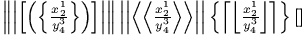(see also here).

Example showing all delimiters


$\left(\left[\left\lbrack \left\{\left\lbrace\left\lfloor \left\lceil\left\langle\left/ \left|\left\|\left\uparrow \left\downarrow\left\updownarrow\left<\left\lmoustache \left\lgroup\frac 12\right\rgroup \right\rmoustache\right>\right\Updownarrow\right\downarrow \right\Uparrow\right\Vert\right\vert \right\backslash\right\rangle \right\rceil\right\rfloor\right\rbrace\right\} \right\rbrack\right]\right)$


The XML translation is

<formula type='display'>
<math mode='display' xmlns='http://www.w3.org/1998/Math/MathML'>
<mfenced open='(' close=')'>
<mfenced open='[' close=']'>
<mfenced open='[' close=']'>
<mfenced open='&lbrace;' close='&rbrace;'>
<mfenced open='&lbrace;' close='&rbrace;'>
<mfenced open='&lfloor;' close='&rfloor;'>
<mfenced open='&lceil;' close='&rceil;'>
<mfenced open='&langle;' close='&rangle;'>
<mfenced open='/' close='&#x2216;'>
<mfenced open='&vert;' close='&vert;'>
<mfenced open='&parallel;' close='&parallel;'>
<mfenced open='&uparrow;' close='&Uparrow;'>
<mfenced open='&downarrow;' close='&downarrow;'>
<mfenced open='&updownarrow;' close='&Updownarrow;'>
<mfenced open='&langle;' close='&rangle;'>
<mfenced open='&lmoustache;' close='&rmoustache;'>
<mfenced separators='' open='&#x3014;' close='&#x3015;'>
<mfrac><mn>1</mn> <mn>2</mn></mfrac>
</mfenced></mfenced></mfenced></mfenced></mfenced></mfenced></mfenced>
</mfenced></mfenced></mfenced></mfenced></mfenced></mfenced></mfenced>
</mfenced></mfenced></mfenced>
[/itex]
</formula>
`

## \Vert, \@Vert (math symbol)

The \Vert command is valid only in math mode. It generates a delimiter (double vertical bar). The character associated to the delimiter is &parallel;, Unicode U+2225, ∥. The \@Vert produces &verbar; Unicode U+2016, ‖ this is a variant.See description of the \vert command.

## \vfil

According to Knuth, \vfil is essentially equivalent to \vskip 0pt plus 1 fil. In Tralics, this command terminates the current paragraph and inserts a <vfil/> element.

## \vfill

According to Knuth, \vfill is essentially equivalent to \vskip 0pt plus 1 fill. In Tralics, this command terminates the current paragraph and inserts a <vfill/> element.

## \vfilneg

In Tralics, this command terminates the current paragraph and inserts a <vfilneg/> element.

## \vfuzz (rigid length)

You can say \vfuzz=5pt, as a result TeX will complain about overfull vboxes only if they are overful by more than 5pt. This is set to 0.1pt by LaTeX, and to .5pt by \sloppy. Ignored by Tralics.(See scandimen for details of argument scanning).

## \voidb@x (constant)

The value of the command is a reference to a box register, that should be kept empty. If you \unhbox\voidb@x, the following happens: in vertical mode, a new paragraph is started (this may add a paragraph indentation, as well as the tokens in \everypar); after that, since the box is empty, nothing happens. This is how \leavevmode is implemented in LaTeX.

## \@vpt, @vipt, \@viipt, \@viiipt (constant)

These four commands expand to 5, 6, 7 and 8 respectively.

## \@vobeyspaces

This command makes the space character active, the expansion being \nobreakspace. Note that \obeyspaces makes the space an active character, it assumes that the translation of a space is \space, hence \@vobeyspaces should be used only in a group.

## \voffset (rigid length)

You can say \voffset=.5in, as a result TeX will shift by .5in vertically the box put by \shipout in the dvi file. Not used by Tralics.(See scandimen for details of argument scanning).

See \hphantom.

See \hrule.

## \vsize (rigid length)

You can say \vsize=15in, as a result TeX will use this quantity as the page height. Ignored by Tralics. In LaTeX, the document class should specify \textheight, from which \vsize is deduced. A LaTeX document should neither access nor modify the \vsize variable.(See scandimen for details of argument scanning).

## \vskip, \vglue

The \vskip command takes a glue value as argument, for instance \vskip 15pt plus 2 fill. Only the fixed part of the glue is considered. It is adds spacebefore='15.0pt' as attribute to the current paragraph. The \vglue command is a bit more complicated in LateX, but implemented in the same way in Tralics.

If the configuration file contains att_space_before = "foo" then foo is used instead of spacebefore as attribute name. The same can be achieved by saying \ChangeElementName*{space_before}{foo}.

If Tralics is in vertical mode, a new paragraph is started (and this paragraph gets the attribute). If it is in horizontal mode, and the paragraph is not empty, a new paragraph is started. Otherwise, if the paragraph has already a spacebefore attribute, the sum of the values is considered.

Note that \\[1cm]\\[2cm] creates two paragraphs. On the other hand, the commands \bigskip \medskip and \smallskip call \vskip, so that \smallskip\smallskip is the same as \medskip.

## \vspace

The translation of \vspace{xx} or \vspace*{xx} is the same as \vskip xx\relax. See above.

## \vsplit

If you say \setbox0=\vsplit 25 to 4cm, then box0 is set to a vbox of height 4cm; it is formed by some material taken off from box 25, using a least-cost algorithm. What happens in Tralics is that nothing is moved. Said otherwise, box25 is left unchanged, and the box produced by \vsplit is empty.See \vbox

## \vss

The \vss command is like \vfil, but inserts a <vss/> element.

## \vtop

This is the same as \vbox.

## \Vvdash (math symbol)

The \Vvdash command is like \vdash, it produces character U+22AA, ⊪; there is a triple vertical bar.back to home page © INRIA 2003-2005, 2006 Last modified $Date: 2015/12/01 17:24:43$